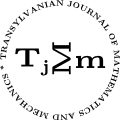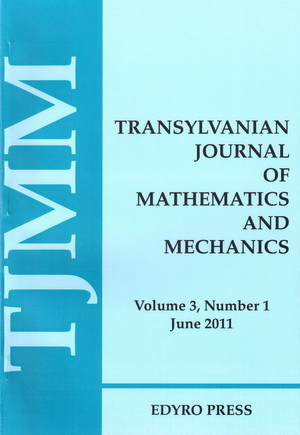ISSN: 2067-239X
ISSN(on-line): 2067-239X

Indexed in:
Mathematical Reviews
Zentralblatt MATH
EBSCO

Front cover# Volume 3 (2011), Number 2

## On Some New Fejér-type Inequalities for Co-ordinated Convex Functions

### Author(s): M. A. LATIF, S. HUSSAIN AND S. S. DRAGOMIR

Abstract: In this paper some new mappings associated with the Fejér inequality for double integrals are deﬁned and as a consequence some new Fejér type inequalities for co-ordinated convex functions are established as well.

## Inequalities of Ostrowski and Simpson Type for Mappings of Two Variables with Bounded Variation and Applications

### Author(s): Y. JAWARNEH AND M.S.M. NOORANI

Abstract: In this paper two inequalities of Ostrowski type and Simpson type for mappings of two independent variables with bounded variation are established. General inequality of trapezoid type is obtained. Applications to some special means and to a quadrature formula are given.

## Generalized Type of Entire Monogenic Functions of Slow Growth

### Author(s): SUSHEEL KUMAR AND KIRANDEEP BALA

Abstract: In the present paper we study the generalized growth of entire monogenic functions having slow growth. The characterizations of generalized type of entire monogenic functions have been obtained in terms of their Taylor’s series coefficients.

## On the Best Polynomial Approximation of Generalized Biaxisymmetric Potentials in ${L}^{p}$-norm, $p\ge 1$

### Author(s): HUZOOR H. KHAN AND RIFAQAT ALI

Abstract: The real valued regular solution of generalized biaxially symmetric potential equation $\frac{{\partial }^{2}F}{\partial {x}^{2}}+\frac{{\partial }^{2}F}{\partial {y}^{2}}+\frac{\left(2\alpha +1\right)}{x}\frac{\partial F}{\partial x}+\frac{\left(2\beta +1\right)}{y}\frac{\partial F}{\partial y}=0,\alpha >\beta >-\frac{1}{2}$ are called generalized biaxisymmetric potentials. In this paper, the characterization of lower order and lower type of entire GBASP $F$ in term of their approximation error ${E}_{n}^{p}\left({F}_{\sigma }\right)$ in ${L}^{p}$-norm, $p\ge 1$ have been obtained. The analysis utilizes the Bergman and Gilbert Integral Operator Method to extend results from classical function theory on the best polynomial approximation of analytic functions of one complex variable.

## On $q$-Hahn’s Theorem: a $q$-extension

### Author(s): L. KHÉRIJI AND P. MARONI

Abstract: We give a $q$-analogue of Hahn’s theorem and a $q$-extension for it; we show that an orthogonal polynomial sequence, whose $m-th$ associated sequence of $k-th$ $q$-derivative sequence is orthogonal, is necessarily a ${H}_{q}$-classical one, where ${H}_{q}$ is the $q$ -difference operator.

## The Direct and the Inverse Problem for Simple Regression Models

### Author(s): FELICIA ZĂVOIANU AND CONSTANTIN ZĂVOIANU

Abstract: This article contains a personal point of view regarding the implementation of simple regression models. We consider that the approach of considering in parallel both the direct and inverse problems helps in constructing a global, in depth, image regarding the usefulness of simple regression problems.

## Study of Improvement of Rotation Mechanism Ball of EsRc-1400 Rotor Excavator

### Author(s): VILHELM ITU, IOSIF DUMITRESCU AND DUMITRU JULA

Abstract: The paper presents the study of the possibility of improvement of EsRc-1400 rotor excavator’s rotation mechanism ball.